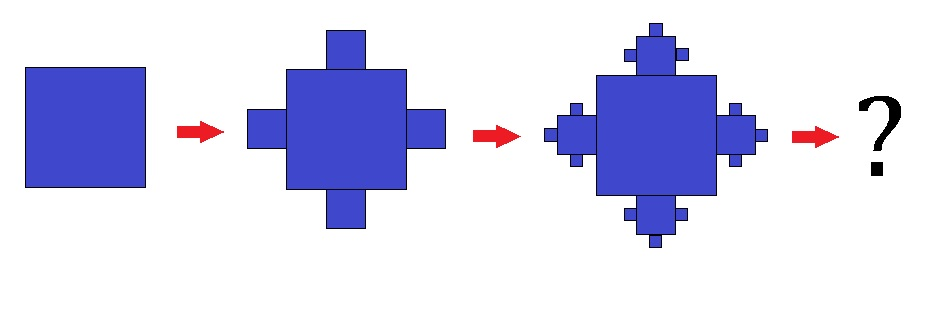# Fractal Squares

Algebra Level 2The image above shows a construction of fractal by joining smaller and smaller squares to each side of one single square.

• We start with a square of side length 3.
• In the second figure, the 4 new squares are formed with a side length of $$\dfrac{1}{3}$$ of its previous square.
• Then from the third figure and so on, the new squares can only be formed on the 3 sides of the previous squares with side length $$\dfrac{1}{3}$$ of its previous square.

As this recursion continues indefinitely, what does the total area of the figure tend to?

×

Problem Loading...

Note Loading...

Set Loading...